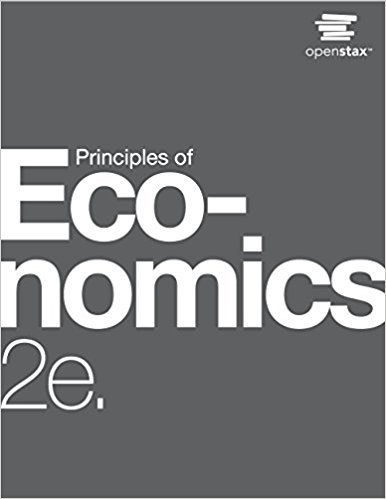×
Get Full Access to Principles Of Economics - 2 Edition - Chapter 22 - Problem 3
Get Full Access to Principles Of Economics - 2 Edition - Chapter 22 - Problem 3

×

# Solved: Compute the inflation rate for fruit prices from 2001 to 2004ISBN: 9781947172364 471

## Solution for problem 3 Chapter 22

Principles of Economics | 2nd Edition

• Textbook Solutions
• 2901 Step-by-step solutions solved by professors and subject experts
• Get 24/7 help from StudySoup virtual teaching assistantsPrinciples of Economics | 2nd Edition

4 5 1 267 Reviews
31
3
Problem 3

Compute the inflation rate for fruit prices from 2001 to 2004.

Step-by-Step Solution:
Step 1 of 3

Chapter 6: Individual and Market Demand Sections: 6.1, 6.2, 6.3, 6.4, 6.5, 6.6 Section 6.1: Individual Demand Price Changes When the price of a good changes, the amount demanded does not change, but the quantity demanded/quantity consumed changes If the price increases, the QD decreases If the price decreases, the QD increases The Individual Demand Curve Price consumption curve: curve tracing the utility maximizing combinations of two goods as the price of one changes Individual demand curve: curve relating the quantity of a good that a single consumer will buy to its price; has two important properties: 1. The level of utility that can be attained changes as we move along the curve. The lower the price of the product, the higher the level of ut

Step 2 of 3

Step 3 of 3

##### ISBN: 9781947172364

This full solution covers the following key subjects: . This expansive textbook survival guide covers 37 chapters, and 1291 solutions. This textbook survival guide was created for the textbook: Principles of Economics, edition: 2. The answer to “Compute the inflation rate for fruit prices from 2001 to 2004.” is broken down into a number of easy to follow steps, and 11 words. Since the solution to 3 from 22 chapter was answered, more than 232 students have viewed the full step-by-step answer. The full step-by-step solution to problem: 3 from chapter: 22 was answered by , our top Business solution expert on 03/16/18, 04:24PM. Principles of Economics was written by and is associated to the ISBN: 9781947172364.

Unlock Textbook Solution